# High School Math : Finding Asymptotes

## Example Questions

### Example Question #1 : Finding Asymptotes

A function is defined by the following rational equation: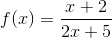What are the horizontal and vertical asymptotes of this function's graph?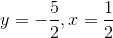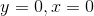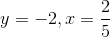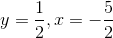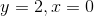Explanation:

To find the horizontal asymptote, compare the degrees of the top and bottom polynomials. In this case, the two degrees are the same (1), which means that the equation of the horizontal asymptote is equal to the ratio of the leading coefficients (top : bottom). Since the numerator's leading coefficient is 1, and the denominator's leading coefficient is 2, the equation of the horizontal asymptote is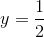.

To find the vertical asymptote, set the denominator equal to zero to find when the entire function is undefined: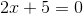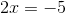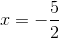### Example Question #2 : Finding Asymptotes

A function is defined by the following rational equation: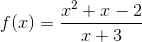What line doesapproach asapproaches infinity?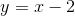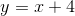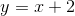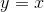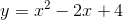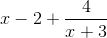However, because we are consideringas it approaches infinity, the effect that the last term has on the overall linear equation quickly becomes negligible (tends to zero). Thus, it can be ignored.